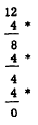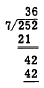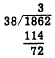Custom SearchPlacement of Decimal Points In any whole number in the decimal system, there is understood to be a terminating mark, called a decimal point, at the right-hand end of the number. Although the decimal point is seldom shown except in numbers involving decimal fractions (covered in chapter 5 of this course), its location must be known. The placement of the decimal point is automatically taken care of when the end Os are correctly placed. Practice problems. Multiply in each of the following problems:DIVISION METHODS Just as multiplication can be considered as repeated addition, division can be considered as repeated subtraction. For example, if we wish to divide 12 by 4 we may subtract 4 from 12 in successive steps and tally the number of times that the subtraction is performed, as follows:As indicated by the asterisks used as tally marks, 4 has been subtracted 3 times. This result is sometimes described by saying that "4 is contained in 12 three times." Since successive subtraction is too cumbersome for rapid, concise calculation, methods which treat division as the inverse of multiplication are more useful. Knowledge of the multiplication tables should lead us to an answer for a problem such as 12 + 4 immediately, since we know that 3 x 4 is 12. However, a problem such as 84 + 4 is not so easy to solve by direct reference to the multiplication table. One way to divide 84 by 4 is to note that 84 is the same as 80 plus 4. Thus 84 + 4 is the same as 80 + 4 plus 4 + 4. In symbols, this can be indicated as follows:(When this type of division symbol is used, the quotient is written above the vinculum as shown.) Thus, 84 divided by 4 is 21. From the foregoing example, it can be seen that the regrouping is useful in division as well as in multiplication. However, the mechanical procedure used in division does not include writing down the regrouped form of the dividend. After becoming familiar with the process, we find that the division can be performed directly, one digit at a time, with the regrouping taking place mentally. The following example illustrates this:The thought process is as follows: "4 is contained in 5 once" (write 1 in tens place over the 5); "one times 4 is 4" (write 4 in tens place under 5, take the difference, and bring down 6); and "4 is contained in 16 four times" (write 4 in units place over the 6). After a little practice, many people can do the work shown under the dividend mentally and write only the quotient, if the divisor has only 1 digit. The divisor is sometimes too large to be contained in the first digit of the dividend. The following example illustrates a problem of this kind:Since 2 is not large enough to contain 7, we divide 7 into the number formed by the first two digits, 25. Seven is contained 3 times in 25; we write 3 above the 5 of the dividend. Multiplying, 3 times 7 is 21; we write 21 below the first two digits of the dividend. Subtracting, 25 minus 21 is 4; we write down the 4 and bring down the 2 in the units place of the dividend. We have now formed a new dividend, 42. Seven is contained 6 times in 42; we write 6 above the 2 of the dividend. Multiplying as before, 6 times 7 is 42; we write this product below the dividend 42. Subtracting, we have nothing left and the division is complete. Estimation When there are two or more digits in the divisor, it is not always easy to determine the first digit of the quotient. An estimate must be made, and the resulting trial quotient may be too large or too small. For example, if 1,862 is to be divided by 38, we might estimate that 38 is contained 5 times in 186 and the first digit of our trial divisor would be 5. However, multiplication reveals that the product of 5 and 38 is larger than 186. Thus we would change the 5 in our quotient to 4, and the problem would then appear as follows:On the other hand, suppose that we had estimated that 38 is contained in 186 only 3 times. We would then have the following:Now, before we make any further moves in the division process, it should be obvious that something is wrong. If our new dividend is large enough to contain the divisor before bringing down a digit from the original dividend, then the trial quotient should have been larger. In other words, our estimate is too small. Proficiency in estimating trial quotients is gained through practice and familiarity with number combinations. For example, after a little experience we realize that a close estimate can be made in the foregoing problem by thinking of 38 as "almost 40." It is easy to see that 40 is contained 4 times in 186, since 4 times 40 is 160. Also, since 5 times 40 is 200, we are reasonably certain that 5 is too large for our trial divisor.Integrated Publishing, Inc. - A (SDVOSB) Service Disabled Veteran Owned Small Business